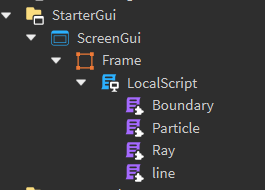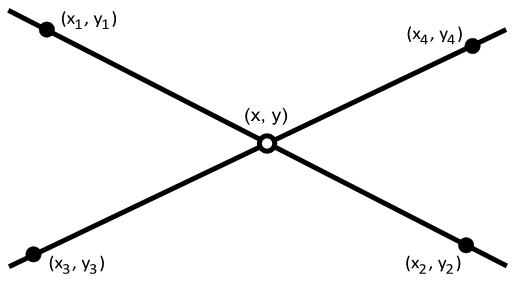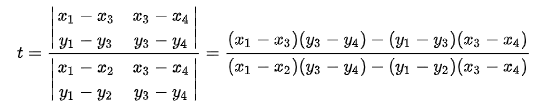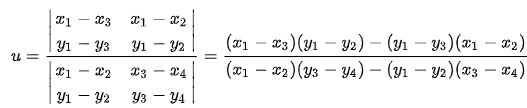# Implementing 2D raycasting!

Hello developers!

I’ve recently implemented 2D raycasting using GuiObjects and other developers wanted a tutorial so here it is!

Post where I showcased this system

NOTE: Some OOP was used in this project so a solid understanding of OOP can help.

So, without further to do, let’s get started!
Let’s start of by creating our project structure, it will be:

• ScreenGui
• Frame
• LocalScript
• Ray ModuleScript
• Boundary ModuleScript
• Particle ModuleScript
• line ModuleScript (just a function)

Your structure should now look like this:Now, our main class are:

1. Ray
2. Boundary (walls)
3. Particle (holder for the rays)

the function `line` will be the easiest to do so let’s do that first!

``````local parent = script.Parent.Parent

local function draw(length, frame)

end

return function (originX, originY, endPointX, endPointY, frame)

end
``````

In the returned function…

Let’s create a vector for both origin and endpoint as well as the net vector

``````local origin = Vector2.new(originX, originY)
local endPoint = Vector2.new(endPointX, endPointY)
local netVector = endPoint - origin
``````

We need to calculate the length, position and angle of the frame so, here is the code:

``````local length = math.sqrt(netVector.X ^ 2 + netVector.Y ^ 2)
local midpoint = Vector2.new((origin.X + endPoint.X) / 2, (origin.Y + endPoint.Y) / 2)
local theta = math.deg(math.atan2(originY - endPointY, originX - endPointX))
``````

All this code is pure mathematics so knowing trignomentry and some basic graph rules will help you understand them!

Let’s now draw the line and give it correct length, position and rotation and return it.

``````local line = draw(length, frame)
line.Position = UDim2.fromOffset(midpoint.X, midpoint.Y)
line.Rotation = theta

return line
``````

Now, the main function is done, let’s make the draw function, it just creates a frame, sets it’s size and changes the appropriate properties

``````local function draw(length, frame)
local line = frame or Instance.new("Frame")
line.Name = "line"
line.AnchorPoint = Vector2.new(.5, .5)
line.Size = UDim2.new(0, length, 0, 2)
line.BackgroundColor3 = Color3.fromRGB(255, 255, 255)
line.BorderSizePixel = 0
line.ZIndex = 1
line.Parent = parent

return line
end
``````

You may have noticed that there’s a parameter called frame, this is for performance! The rays’ position changes every frame so we can’t create new GuiObjects every frame, that will be a disaster!

Our `line` ModuleScript code should look like this now

``````local parent = script.Parent.Parent

local function draw(length, frame)
local line = frame or Instance.new("Frame")
line.Name = "line"
line.AnchorPoint = Vector2.new(.5, .5)
line.Size = UDim2.new(0, length, 0, 2)
line.BackgroundColor3 = Color3.fromRGB(255, 255, 255)
line.BorderSizePixel = 0
line.ZIndex = 1
line.Parent = parent

return line
end

return function (originX, originY, endPointX, endPointY, frame)
local origin = Vector2.new(originX, originY)
local endPoint = Vector2.new(endPointX, endPointY)
local netVector = endPoint - origin

local length = math.sqrt(netVector.X ^ 2 + netVector.Y ^ 2)
local midpoint = Vector2.new((origin.X + endPoint.X) / 2, (origin.Y + endPoint.Y) / 2)
local theta = math.deg(math.atan2(originY - endPointY, originX - endPointX))

local line = draw(length, frame)
line.Position = UDim2.fromOffset(midpoint.X, midpoint.Y)
line.Rotation = theta

return line
end
``````

Now, let’s create the actual classes, let’s start with the simplest one the Boundary class.

# Boundary Class

(Boundary ModuleScript)
We will start off by making a Boundary class

``````local Boundary = {}
Boundary.__index = Boundary

function Boundary.new(x1, y1, x2, y2)
return setmetatable({}, Boundary)
end

return Boundary
``````

Let’s add the needed items to the `.new` function:

``````function Boundary.new(x1, y1, x2, y2)
return setmetatable({
a = Vector2.new(x1, y1),
b = Vector2.new(x2, y2),
frame = line(x1, y1, x2, y2)
}, Boundary)
end
``````

Let’s require our `line` module:

``````local line = require(script.Parent.line)
``````

``````function Boundary:Show()

end

function Boundary:Remove()

end
``````

Now let’s make them functional!

``````function Boundary:Show()
self.frame = line(self.a.X, self.a.Y, self.b.X, self.b.Y, self.frame)
end

function Boundary:Remove()
self.frame:Destroy()
end
``````

Just simply drawing and destroying the boundary.

Your Boundary class should now look like this:

``````local Boundary = {}
Boundary.__index = Boundary

local line = require(script.Parent.line)

function Boundary.new(x1, y1, x2, y2)
return setmetatable({
a = Vector2.new(x1, y1),
b = Vector2.new(x2, y2),
frame = line(x1, y1, x2, y2)
}, Boundary)
end

function Boundary:Show()
self.frame = line(self.a.X, self.a.Y, self.b.X, self.b.Y, self.frame)
end

function Boundary:Remove()
self.frame:Destroy()
end

return Boundary
``````

And voila! You got a boundary class! 2 more classes to go!

## Ray Class

We will start of by making the class, just like in Boundary, I’ll include the main functions and complete the `.new()` as there’s nothing too interesting going on.

``````local Ray = {}
Ray.__index = Ray

local line = require(script.Parent.line)

function Ray.new(position, angle)
return setmetatable({
position = position,
direction = Vector2.new(math.cos(angle), math.sin(angle)).Unit
}, Ray)
end

function Ray:LookAt(x, y)

end

function Ray:Show()

end

function Ray:SetPosition()

end

function Ray:Cast()

end

return Ray
``````

Time to make these functions do something, more math!
The `LookAt` function is pretty simple so let’s do it:

``````function Ray:LookAt(x, y)
self.direction = Vector2.new(x - self.position.X, y - self.position.Y).Unit
end
``````

We use `.Unit` as we are setting the direction which needs to be a ray with magnitude of 1
Next! The `Show` and `SetPosition` functions are simple and self explanatory so here they are:

``````function Ray:Show()
self.frame = line(self.position.X, self.position.Y, self.position.X + self.direction.X * 10, self.position.Y + self.direction.Y * 10, self.frame)
end

function Ray:SetPosition(position)
self.position = position
end
``````

Now for the fun part! The `Cast` method, concentrate now, full concentration and let’s go!
Since we are working with line segments, we will need some function that takes in x1, x2, x3, x4, y1, y2, y3, y4 and returns x, y (look at image down)The cast function will do exactly what we need! Here are the formulas:WHAT!, I hear you screaming right there! Jokes aside, I know it looks scary but let’s break it down a bit, if you look closely, the denominator of both fractions is exactly the same! That saves us a lot of heart break formula writing! Wait wait wait wait, before we start, what are x1, x2, x3, x4, y1, y2, y3 and y4, man that’s a lot of symbols! Let’s make variables for them so we don’t get lost when writing the formula!

``````local x1, y1 = wall.a.X, wall.a.Y
local x2, y2 = wall.b.X, wall.b.Y
local x3, y3 = self.position.X, self.position.Y
local x4, y4 = self.position.X + self.direction.X, self.position.Y + self.direction.Y
``````

Now, time for the tedious formula! WAIT! Do you remember what we said about the denominators? Yes, exactly, they are the same, so, let’s make a variable for it

``````local denominator = (x1 - x2) * (y3 - y4) - (y1 - y2) * (x3 - x4)
if denominator == 0 then return end
``````

Now you may ask, why are we checking if it’s 0 and stopping the function there? Well, `n / 0` will result in `inf` which will ruin the calculations! We can’t let that pass. Time for the actual formula now, it will be a bit more simplified as we made a “helper” variable

``````local t = ((x1 - x3) * (y3 - y4) - (y1 - y3) * (x3 - x4)) / denominator
local u = ((x1 - x2) * (y1 - y3) - (y1 - y2) * (x1 - x3)) / denominator
``````

There’s a problem in the formula, can you find it?
hint: It’s in the second formula.
answer: We forgot the negative sign! Ah yes, my worst enemy!
Here’s the corrected code:

``````local t = ((x1 - x3) * (y3 - y4) - (y1 - y3) * (x3 - x4)) / denominator
local u = -((x1 - x2) * (y1 - y3) - (y1 - y2) * (x1 - x3)) / denominator
``````

Now, let’s make an if statement for the inequalities above:

``````if t < 0 or t > 1 or u < 0 then return end
``````

Let’s now return the point of intersection:

``````return Vector2.new(
x1 + t * (x2 - x1),
y1 + t * (y2 - y1)
)
``````

Finally! Done! Your Ray class should look like this:

``````local Ray = {}
Ray.__index = Ray

local line = require(script.Parent.line)

function Ray.new(position, angle)
return setmetatable({
position = position,
direction = Vector2.new(math.cos(angle), math.sin(angle)).Unit
}, Ray)
end

function Ray:LookAt(x, y)
self.direction = Vector2.new(x - self.position.X, y - self.position.Y).Unit
end

function Ray:Show()
self.frame = line(self.position.X, self.position.Y, self.position.X + self.direction.X * 10, self.position.Y + self.direction.Y * 10, self.frame)
end

function Ray:SetPosition(position)
self.position = position
end

function Ray:Cast(wall)
local x1, y1 = wall.a.X, wall.a.Y
local x2, y2 = wall.b.X, wall.b.Y
local x3, y3 = self.position.X, self.position.Y
local x4, y4 = self.position.X + self.direction.X, self.position.Y + self.direction.Y

local denominator = (x1 - x2) * (y3 - y4) - (y1 - y2) * (x3 - x4)
if denominator == 0 then return end

local t = ((x1 - x3) * (y3 - y4) - (y1 - y3) * (x3 - x4)) / denominator
local u = -((x1 - x2) * (y1 - y3) - (y1 - y2) * (x1 - x3)) / denominator

if t < 0 or t > 1 or u < 0 then return end

return Vector2.new(
x1 + t * (x2 - x1),
y1 + t * (y2 - y1)
)
end

return Ray
``````

# Particle Class

Same start, making the class, completing the `.new()` function and putting the needed functions

``````local Particle = {}
Particle.__index = Particle

local line = require(script.Parent.line)
local Ray = require(script.Parent.Ray)

local frame = script.Parent.Parent
local size = frame.AbsoluteSize

local ANGLE_DIFFERENCE = 5

function Particle.new()
local position = size / 2
local rays = {}

for angle = 0, 360, ANGLE_DIFFERENCE do
end

return setmetatable({
position = position,
rays = rays,
rayLines = {}
}, Particle)
end

function Particle:Update()

end

function Particle:Look()

end

function Particle:Show()

end

return Particle
``````

That’s a lot of stuff, what is all of that? Well, it just loops through all the 360 degrees and increments bye `ANGLE_DIFFERENCE` constant, there are some variables but I think they are self explanatory.
The update function is just to set the position so:

``````function Particle:Update(x, y)
self.position = Vector2.new(x, y)
end
``````

then the `Show` function, it hyst draws all the rays and changes their positions to avoid wrong intersection point calculation:

``````function Particle:Show()
for _, ray in ipairs(self.rays) do
ray:SetPosition(self.position)
ray:Show()
end
end
``````

Now for the `Look` method, the most important one:

``````function Particle:Look(walls)
for i, ray in ipairs(self.rays) do
local closest = nil
local record = math.huge

ray:SetPosition(self.position)

for _, wall in ipairs(walls) do
local point = ray:Cast(wall)
if not point then continue end

local distance = (self.position - point).Magnitude
if distance > record then continue end

record = distance
closest = point
end

if closest then
self.rayLines[i] = line(self.position.X, self.position.Y, closest.X, closest.Y, self.rayLines[i])
end
end
end
``````

Let’s break it down, we first loop through all rays, then create 2 variables, `closest` for closest point and `record` for distances and set the ray’s position to avoid incorrect calculations. Then we loop through all the walls and cast our ray, in case it hit something, `point` will be returned, and we will calculate the distance, if the distance is closer than `record` (meaning it’s closer to the ray), we will set it as the closest point and change record to the new distance. After all the loops, we check if there is a `closest` point, meaning the ray hit something, if there is, draw a line and save it inside an array, to avoid creating new lines (performance!).

Now, you are done! Let's make a quick code to run this system and view it's beauty! Inside the LocalScript:
``````local runService = game:GetService("RunService")
local players = game:GetService("Players")
local userInputService = game:GetService("UserInputService")

local player = players.LocalPlayer
local mouse = player:GetMouse()

local Particle = require(script.Particle)
local Boundary = require(script.Boundary)

local frame = script.Parent
local size = frame.AbsoluteSize
local width = size.X
local height = size.Y

local walls = {}
local particle = Particle.new()

local NUMBER_OF_WALLS = 10

local function generateWalls()
for i, wall in pairs(walls) do
wall:Remove()
end

table.clear(walls)

for i = 1, NUMBER_OF_WALLS do
local x1 = math.random(width)
local x2 = math.random(width)
local y1 = math.random(height)
local y2 = math.random(height)

walls[i] = Boundary.new(x1, y1, x2, y2)
end

table.insert(walls, Boundary.new(0, 0, width, 0))
table.insert(walls, Boundary.new(width, 0, width, height))
table.insert(walls, Boundary.new(width, height, 0, height))
table.insert(walls, Boundary.new(0, height, 0, 0))
end

generateWalls()

runService.RenderStepped:Connect(function()
particle:Update(mouse.X, mouse.Y)
particle:Look(walls)
end)

userInputService.InputBegan:Connect(function(input, gameProcessedEvent)
if gameProcessedEvent then return end
if input.KeyCode ~= Enum.KeyCode.R then return end

generateWalls()
end)
``````

Let’s explain it! We first make variables for the needed services as well as the player and his mouse (for navigation), then we require the `Particle` and `Boundary` classes we just created and make variables for the width and height of the holder frame. Then we create a table to hold all our walls (Boundaries) and a particle as well as a constant for the number of walls (excluding the borders!)
Then we make a function to generate random walls and then draws the borders and add all of them to the walls table, make sure to clear the table after deleting it to avoid the ray casting to removed objects! We call the function to make some walls for us.
Then, inside the `RenderStepped`, we update our particle’s position and make it cast the rays and draw the ones that hit something.
Lastly we add an `InputBegan` event, just to be able to reset the walls while in the game when you click the button `R`.

And that’s it! You got a (simple) working 2D raycasting system!
If you have any questions, feel free to ask me!

- With love, by Msix.

6 Likes

If you saw any mistakes please inform me.

Why do you need 2 line module scripts? Other than that nice tutorial.

Small mistake, thanks for informing me.

1 Like

you spelled “line” incorrectly

Thank you! I noticed some other miss spells, it’s hopefully all good now.